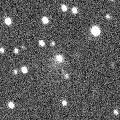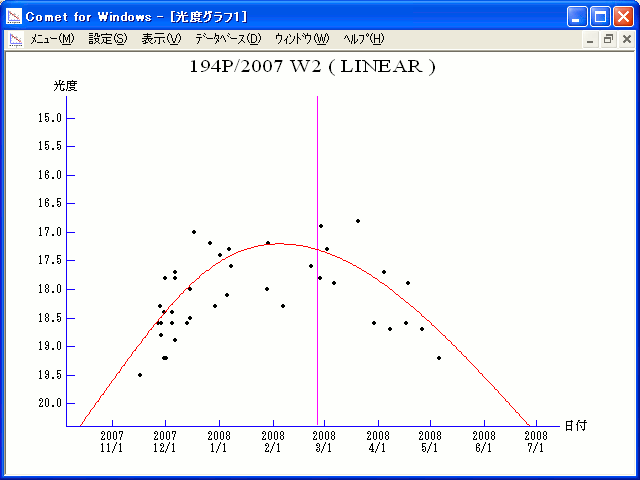# \$B%j%K%"WB@1(B

194P/LINEAR (2008)

 English version Home page Updated on October 12, 2008###\$B%W%m%U%#!<%k(B

 \$BId9f(B 194P/2007 W2 \$B8!=PF|(B 2007\$BG/(B11\$B7n(B17\$BF|(B \$B8!=P8wEY(B 19.5\$BEy(B \$B8!=P L. Buzzi and F. Luppi (Varese, Italy)

###\$B###\$B50F;MWAG(B

```                    Epoch = 2008 Feb. 24.0 TT
T = 2008 Feb. 26.1914 TT         Peri. = 130.6410
e = 0.574210                     Node  = 352.0642  2000.0
q = 1.708753 AU                  Incl. =  11.1181
a =  4.013132 AU    n = 0.1225967    P =   8.039 years
```

###\$B8wEYJQ2=(B

```        m1 = 12.5 + 5 log\$B&\$(B + 20.0 log r(t - 15)
```##### \$B50F;MWAG\$O!"(BIAUC 8900\$B\$K7G:\\$5\$l\$?\$b\$N\$G\$9!#(B \$B8wEY%0%i%U\$O(BComet for Windows\$B\$G:n@.\$7\$?\$b\$N\$G\$9!#(B